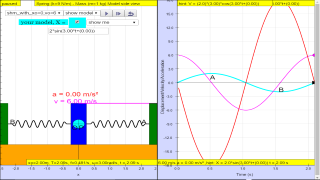SHM11

### 1.2.5 Example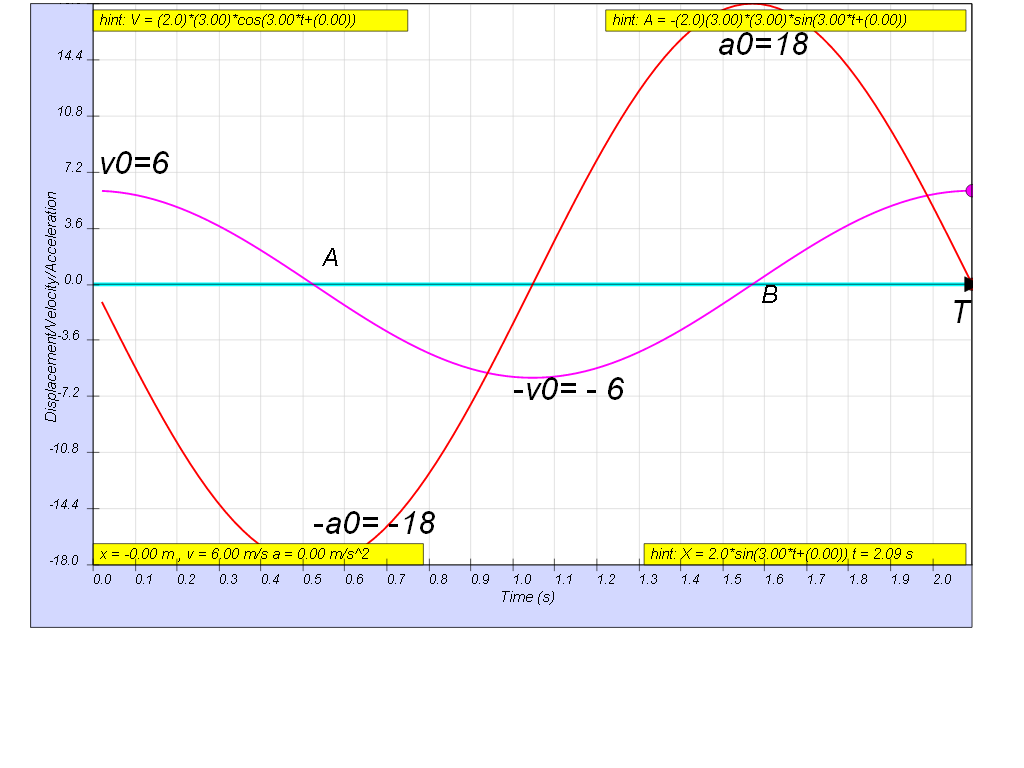The graphs show how the variation with time of the velocity, v and the acceleration, a of a body when it is oscillating with simple harmonic motion.
(a)    Determine the period, T.
(b)    Sketch the variation with time of the displacement, x, of the oscillating body
[ 2.09 s, x = 2  sin( 3t )]

##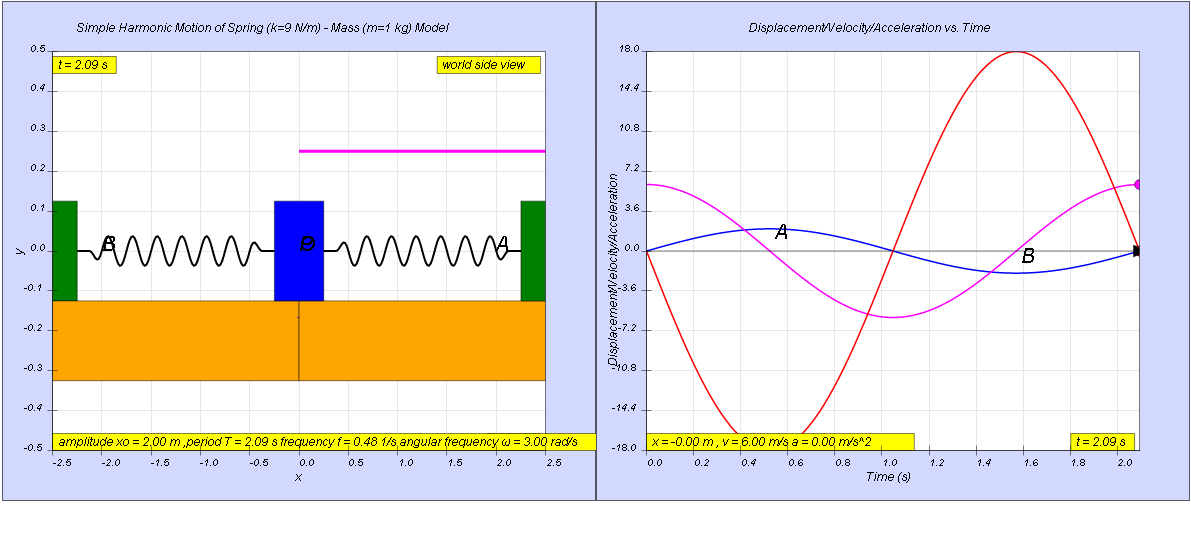### 1.2.5.1 Solution:

a) maximum velocity , v0 = x0ω = 6 m/s

maximum acceleration a0 = x0ω2 = 18 m/s2

thus dividing the 2 equations, we get ω = 3 rad/s

therefore since $\omega =\genfrac{}{}{0.1ex}{}{2\pi }{T}$

$3=\genfrac{}{}{0.1ex}{}{2\pi }{T}$

$T=\genfrac{}{}{0.1ex}{}{2\pi }{3}$

$T=2.09s$

b) since maximum velocity , v0 = x0ω = 6 m/s and we now know ω = 3 rad/s earlier

x0(3) = 6

x0 = 2 m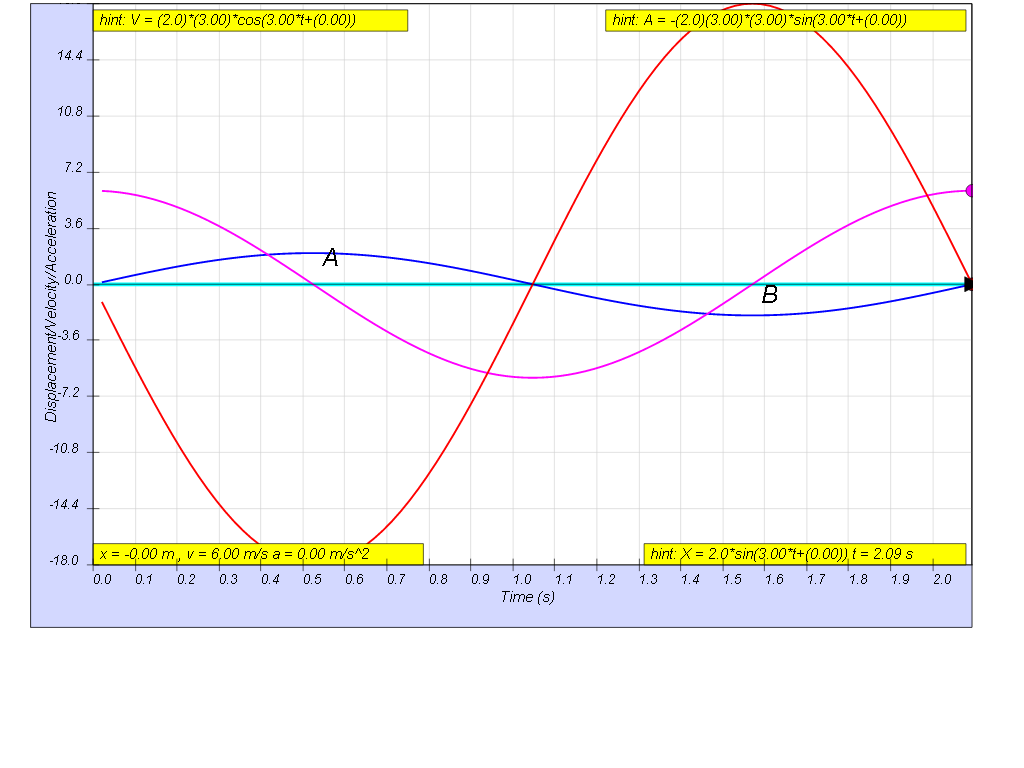### Translations

Code Language Translator Run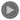### Software Requirements

SoftwareRequirements

 Android iOS Windows MacOS with best with Chrome Chrome Chrome Chrome support full-screen? Yes. Chrome/Opera No. Firefox/ Samsung Internet Not yet Yes Yes cannot work on some mobile browser that don't understand JavaScript such as..... cannot work on Internet Explorer 9 and below

### CreditsThis email address is being protected from spambots. You need JavaScript enabled to view it.

### end faq

http://iwant2study.org/lookangejss/02_newtonianmechanics_8oscillations/ejss_model_SHM11/SHM11_Simulation.xhtml

# Testimonials (0)

There are no testimonials available for viewing. Login to deploy the article and be the first to submit your review!